# Solution July 21, 2007

### Problem

What is the largest positive integer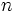$n\,$ such that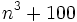$n^3+100\,$ is divisible by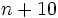$n+10\,$?

### Solution

n + 10 | n3 + 100 is equivalent to gcd(n + 10,n3 + 100) = n + 10 By the rules for the gcd (http://en.wikipedia.org/wiki/Greatest_common_divisor) we have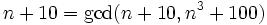$n+10 = \gcd(n+10, n^3+100)\,$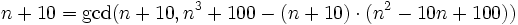$n+10 = \gcd(n+10, n^3+100 - (n+10)\cdot(n^2-10n+100))$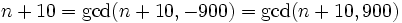$n+10 = \gcd(n+10, -900) = \gcd(n+10, 900)\,$

In other words, n + 10 | 900 and the largest such n is n = 890.